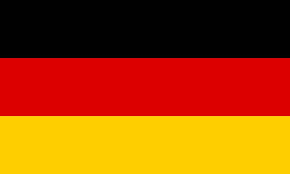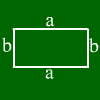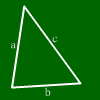# Area calculationEnter two values. Other values will be calculated.Side a: Side b: area Perimeter: diagonal
 This calculator computes the values of a circle (radius, diameter, circumference or area) when one value is given.Fill in one field. The others will be calculated. Radius r: Diameter d: perimeter U: Area A:
 This calculator calculates from three values of a triangle all other values.Enter three values. Other values will be calculated. a: b: c: alpha : beta : gamma : Height: ha: Height: hb: Height: hc: Area Not often needed (let empty if not needed): Median line: sa: sb: sc: Angle bisector: wa: wb: wc:
 Calculate a square Enter one value. Other values will be calculated. Side Area: Perimeter: diagonal

# Calculating a rhomb

Enter two values. Other values will be calculated.Side a:
Diagonal e: Diagonal f:
alpha beta
Perimeter:
Area:

 Enter one function in here. Hint: Enteras 3*x^2 ,as 3/5 andas (x+1)/(x-2x^4) Enter the other function in here. To write powers, use ^. This means, you gotta write x^2 for.

If you got some information about your area, the question is how to calculate the other informations. In many cases this is possible. A square or a circle e.g. are already given by a single information (e.g. perimeter or area). Having areas with less symmetries means we need more information. Mathepower has many area calculors.

Calculators for that topic:
Triangle calculator
Circle calculator
Arc calculator
Rhomboid calculator
Square calculator
Rhomb calculator
Rectangle calculator
Trapezoid calculator